# Thread: Derivative

1. ## Derivative

How do i find the derivative of the following? I'm having trouble on what kind of rule to use.

f(x)=5/(x^3) - 3/(2-7x) + 3x^5 - 15 (Quotient rule?)

g(x) = tan(x)ln(2x+5) (product rule?)

y=cos(3-2x^4) (chain Rule??)

thanks for your help

2.Originally Posted by tim_mannireHow do i find the derivative of the following? I'm having trouble on what kind of rule to use.

f(x)=5/(x^3) - 3/(2-7x) + 3x^5 - 15 (Quotient rule?)
the derivative of a sum is the sum of derivatives. Then the power rule and chainrule.

g(x) = tan(x)ln(2x+5) (product rule?)
product rule and chain rule

y=cos(3-2x^4) (chain Rule??)
chain rule

CB

3. Just in case a picture helps...Originally Posted by CaptainBlackOriginally Posted by tim_mannireHow do i find the derivative of the following? I'm having trouble on what kind of rule to use.

f(x)=5/(x^3) - 3/(2-7x) + 3x^5 - 15 (Quotient rule?)
the derivative of a sum is the sum of derivatives. Then the power rule and chainrule.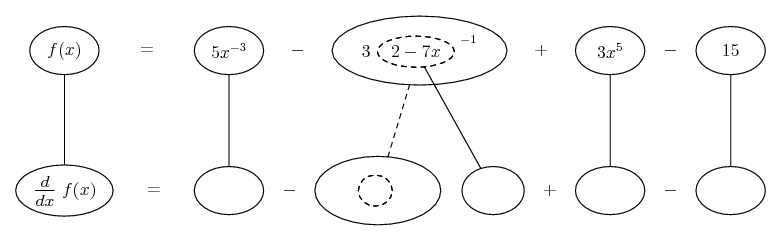Originally Posted by tim_mannireg(x) = tan(x)ln(2x+5) (product rule?)
product rule and chain rule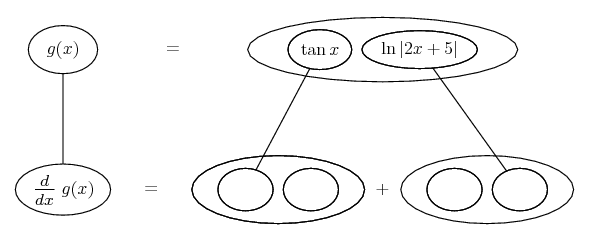... or, zooming in further...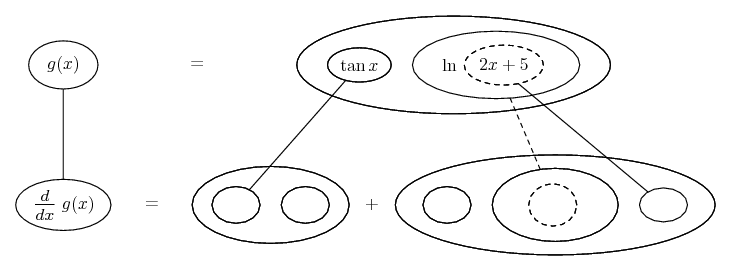Originally Posted by tim_mannirey=cos(3-2x^4) (chain Rule??)

thanks for your help
chain rule

CB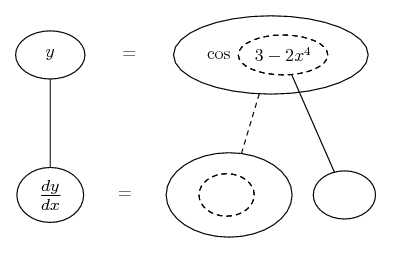... where... is the product rule... the chain rule. Straight continuous lines differentiate downwards (integrate up) with respect to x, and the straight dashed line similarly but with respect to the dashed balloon expression (the inner function of the composite which is subject to the chain rule).

Spoiler: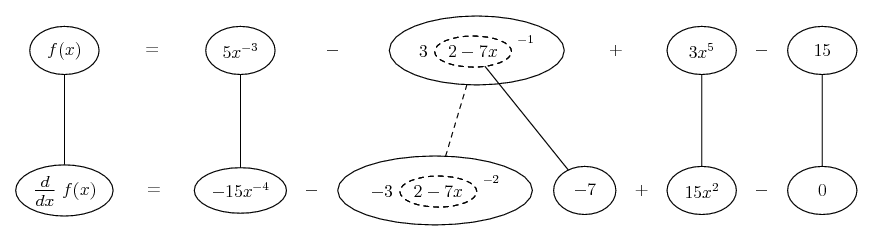Spoiler: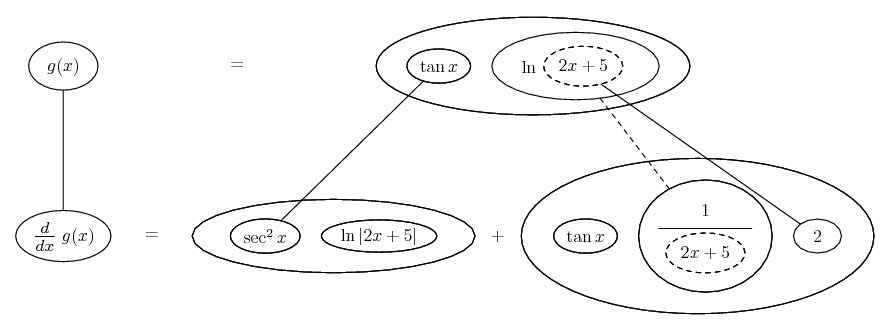Spoiler: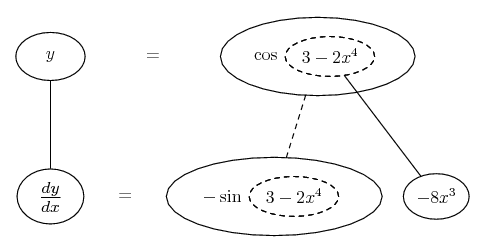_________________________________________

Don't integrate - balloontegrate!

Balloon Calculus; standard integrals, derivatives and methods

Balloon Calculus Drawing with LaTeX and Asymptote!

#### Search Tags

derivative, differentiation